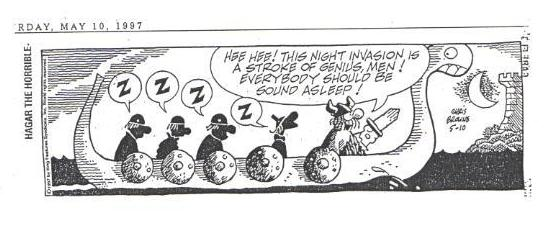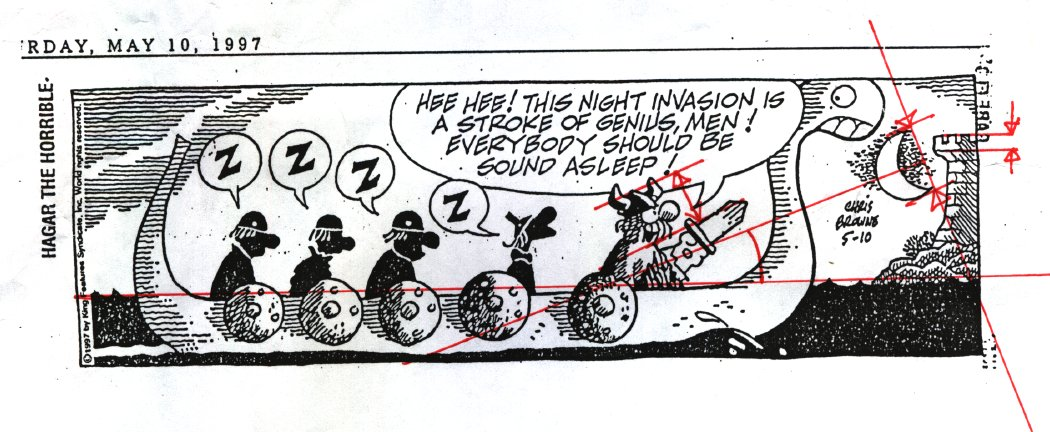Where is Hagar?The clue is the moon. It is half a degree wide (so is the Sun),
which gives us a scale and a measure of distance.

And the moon points toward the sun.

Mark the strip like so:Some measurements; the scale is arbtrary, but when I print out the cartoon, with measurements in centimeters,

```	the moon		1.39 cm
battlement		0.36 cm
moon to horizon 	6.0 cm
the moon is a quarter full
the angle between the horizon and the bisector of the moon
is   20 degrees, or 0.3491 radians
```

The moon gives us a scale, from which distances may be derived, given some assumptions about the size of Hagar's head and the height of the battlement.

The moon at 1.39 cm subtends an angle of a half-degree, or 8.73 x 10^-3 radians, resulting in a conversion factor of 6.278x10^-3 radians per centimeter, 0.36 degrees per centimeter.

From Hagar's beard to the tip of his helmet we will guess is about 12 inches, or 30 cm. So Hagar's head subtends 7.094x10^-3 radians.

Guess that the battlement as depicted measures 10 feet or 3 meters high. The battlement subtends 2.26x10^-3 radians.

To derive the distances, remember that the size d of an object equals the angle subtended times the distance r to the object. Or, rθ = d, and so r = d/θ.

Hagar is thus 42 meters distant; The battlement is 1300 meters distant; plausible, but a stretch, given that the cartoonist probably didn't calculate these scales. The tower is about 50 or 60 feet tall; plausible enough.

What is more interesting is the moon.

The cartoonist has drawn the moon larger than one might expect it to appear in such a scene, effectively moving the castle into the distance.

It is as if the picture were a photograph taken through a telephoto lens.

The limb of the moon nearest the horizon is (6.0 cm) / (2.78 cm = 1 degree) = 2.158 degrees, in the units that are most useful. The moon moves about a degree in four minutes, so the moon arose about ten minutes ago. (Hagar is a Dane, and we assume that we are in the Northern hemisphere, close to his home waters.)

The moon points toward the sun: if both are on the ecliptic, the bright side of the moon is the side toward the sun. The moon is a quarter full. A full moon lies 180 degrees or 12 hours of right ascension from the sun; a half moon 6 hours, a quarter moon 3 hours. We are most of four days into the lunar month. So the sun is 3 hours behind the moon, and has some time still before it rises. We are most of three hours before sunrise, which could be quite early in summertime in northern latitudes where Hagar lives.

Now comes the interesting (and precarious) part: what is our latitude? The moon's orbit is inclined at about 5.5 degrees to the ecliptic, so the line from the moon to the sun is only a hint of where the ecliptic is.

That question will have to wait.